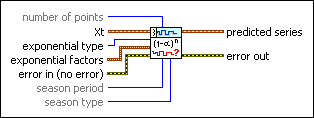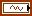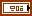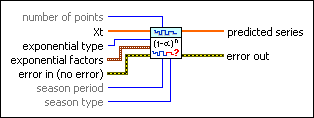# TSA Exponential Prediction VI

LabVIEW 2014 Advanced Signal Processing Toolkit Help

Edition Date: June 2014

Part Number: 372656C-01

»View Product InfoDownload Help (Windows Only)

Owning Palette: Modeling and Prediction VIs

Predicts the values of a univariate time series based on exponential smoothing.

Wire data to the Xt input to determine the polymorphic instance to use or manually select the instance.

Use the pull-down menu to select an instance of this VI.

 Select an instance TSA Exponential Prediction (Waveform)TSA Exponential Prediction (Array)

## TSA Exponential Prediction (Waveform)number of points specifies the length of the predicted time series. The default is 1.Xt specifies the univariate time series.exponential type specifies the type of exponential smoothing scheme to use in the prediction. Refer to the Details section for more information about each exponential type.

 0 Single (default) 1 Double 2 Tripleexponential factors specifies the weighting factors for exponential smoothing.level specifies the weight for the level cumulant. The value of value must be a number between 0 and 1.trend specifies the weight for the trend cumulant. The value of trend must be a number between 0 and 1. This option is available only when exponential type is Double or Triple.season specifies the weight for the seasonal cumulant. The value of season must be a number between 0 and 1. This option is available only when exponential type is Triple.error in describes error conditions that occur before this node runs. This input provides standard error in functionality.season period specifies the length of the seasonal pattern in the input time series. The default is 1. This option is available only when exponential type is Triple.season type specifies the way in which this VI models the seasonality. This option is available only when exponential type is Triple. Refer to the Details section for information about each season type.

 0 Multiplicative (default)—Indicates that the time series has a seasonality with the amplitude increasing over time. 1 Additive—Indicates that the time series has a constant amplitude change in seasonality.predicted series returns the predicted univariate time series.error out contains error information. This output provides standard error out functionality.

## TSA Exponential Prediction (Array)number of points specifies the length of the predicted time series. The default is 1.Xt specifies the univariate time series.exponential type specifies the type of exponential smoothing scheme to use in the prediction. Refer to the Details section for more information about each exponential type.

 0 Single (default) 1 Double 2 Tripleexponential factors specifies the weighting factors for exponential smoothing.level specifies the weight for the level cumulant. The value of value must be a number between 0 and 1.trend specifies the weight for the trend cumulant. The value of trend must be a number between 0 and 1. This option is available only when exponential type is Double or Triple.season specifies the weight for the seasonal cumulant. The value of season must be a number between 0 and 1. This option is available only when exponential type is Triple.error in describes error conditions that occur before this node runs. This input provides standard error in functionality.season period specifies the length of the seasonal pattern in the input time series. The default is 1. This option is available only when exponential type is Triple.season type specifies the way in which this VI models the seasonality. This option is available only when exponential type is Triple. Refer to the Details section for information about each season type.

 0 Multiplicative (default)—Indicates that the time series has a seasonality with the amplitude increasing over time. 1 Additive—Indicates that the time series has a constant amplitude change in seasonality.predicted series returns the predicted univariate time series.error out contains error information. This output provides standard error out functionality.

## TSA Exponential Prediction Details

This VI computes the future values of a time series based on one of the following exponential smoothing schemes: single, double, and triple (Holt-Winters). You can specify the type of exponential smoothing scheme using the exponential type parameter. Each exponential smoothing scheme has a corresponding forecasting formula that uses the computed level cumulant, trend cumulant, and season cumulant vector.

### Single

In the single exponential smoothing scheme, this VI obtains the level cumulant Xa. The corresponding k-step-ahead prediction at time t is defined according to the following equation:

Xt(N + k–1) = Xa(N–1)

where N is the number of original time series Xt.

### Double

In the double exponential smoothing scheme, this VI obtains the level cumulant Xa and trend cumulant B. The corresponding k-step-ahead prediction at time t is defined according to the following equation:

Xt(N+k–1)=Xa(N–1)+kB(N–1)

Refer to the TSA Exponential Average VI for the descriptions of the level cumulant Xa and trend cumulant B.

### Triple

In the triple exponential smoothing scheme, this VI obtains the level cumulant Xa, the trend cumulant B, and the season cumulant vector I=(I1, …, IL). L is seasonal period. If the season type is set as Multiplicative, the corresponding k-step-ahead prediction at time t is defined according to the following equation:

Xt(N+k–1)=(Xa(N–1)+kB(N–1))·It(N+k–1–L)

If the season type is set as Additive, the corresponding k-step-ahead prediction at time t is defined according to the following equation:

Xt(N+k–1)=Xa(N–1)+kB(N–1)+It(N+k–1–L)

## Example

Refer to the Exponential Prediction VI in the labview\examples\Time Series Analysis\TSAGettingStarted directory for an example of using the TSA Exponential Prediction VI.